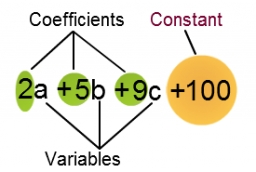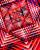# Evaluate expr with 2 vars

If a=6 and x=2, find the value of (2ax + 7x- 10) /(4ax-3a-2)

v =  1

### Step-by-step explanation:Did you find an error or inaccuracy? Feel free to write us. Thank you!Tips to related online calculators
Need help to calculate sum, simplify or multiply fractions? Try our fraction calculator.

## Related math problems and questions:

• Evaluate expressionEvaluate expression using BODMAS rule: 1 1/4+1 1/5÷3/5-5/8
• Two numbers and its productThe product of two numbers are 2/3. If on of them is 1/10, what is the other?
• Evaluate 9Evaluate expression: 3 { 4 − 4 ( 5 − 12 ) } + 20
• Evaluate expressionEvaluate following expression: 5 to the power of 2 - (4x4) divided by 2 =
• Evaluate 12Evaluate and simplify 9 divided by 7/6.
• Expression plus minusEvaluate expression: (-1)2 . 12 – 6 : 3 + (-3) . (-2) + 22 – (-3) . 2
• Evaluate expression 2Evaluate expression with negatives: (-3)+4+(-8)+(-6)+4+(-1)
• Expression with bracketsEvaluate the expression. 15+[(9-4)×22]
• Find unknown 2Find unknown denominator: 2/3 -5/? = 1/4
• Evaluate - order of opsEvaluate the expression: 32+2[5×(24-6)]-48÷24 Pay attention to the order of operation including integers
• The length 6The length of 12 pipes is 10 1/2 meters. (1) find the length of one pipe (2) also find the length of 7 pipes
• Simple sequenceContinue with this series of numbers: 1792,448, 112, _, _
• Two thirdsFind two-thirds of the number equal to two-thirds of 99
• Expression 6Evaluate expression: -6-2(4-8)-9
• TheaterThe theater has in each row with 19 seats. Ticket to the first 10 rows is for 30 USD. In the next rows is for 15 USD. The theater was completely sold out. Revenue was 12255 USD. How many rows are in the theater?
• Evaluate expressionIf x=2, y=-5 and z=3 what is the value of x-2y
• Expression 8Evaluate this expressions: a) 5[3 + 4(2.8 - 3)] b) 5×(8-4)÷4-2